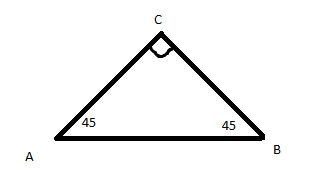# Relatively simple vectors question, but no numbers...

The unit right now is electrostatics, but this question is really just vectors, nothing to do with charges or anything... anyways here is the info:

1. Homework Statement

Three identical point charges, A, B, and C are located as shown here:The force A-on-C is the same as the force B-on-C. What is the net force on C?

## Homework Equations

I think the only equation I need is the superposition of force principle:
FnetC = FAonC + FBonC

## The Attempt at a Solution

[/B]
Breaking into components (not allowed to use cosine law for some reason):

I know X-axis force is 0 as the 2 forces cancel out (since they are both equal and in opposite directions).

FnetCY = FAonC * cos(45) + FBonC * cos(45)

Not sure what else I can do though...

EDIT: I messed around with the 1-1-sqrt(2) triangle and I'm not sure if this is right, but is (2/sqrt(2)) * FAonC the right answer?

EDIT 2: Yes, that's the right answer, but sqrt(2) * FAonC is also right.

Because guess what, 2 divided by squareroot(2) is THE SAME THING AS squareroot(2)...

Last edited:

seems okay to me

assuming the charges on the charges on the corners A and B are all the same polarity

assuming the charges on the charges on the corners A and B are all the same polarity

Yeah they are all identical.

seems okay to me

How would I use the 1-1-squareroot2 triangle to simplify it more? I'm not sure how to do that part too well.

replace your cos45 statement with the equivalent ratio